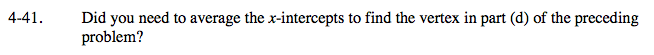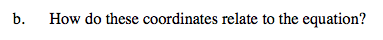### Home > A2C > Chapter 4 > Lesson 4.1.3 > Problem4-41

4-41.
1. Did you need to average the x-intercepts to find the vertex in part (d) of the preceding problem? Homework Help ✎

1. What are the coordinates of the vertex for part (d)?

2. How do these coordinates relate to the equation?(2, −1)Where do they appear in the equation?
What effect do they have on the equation?